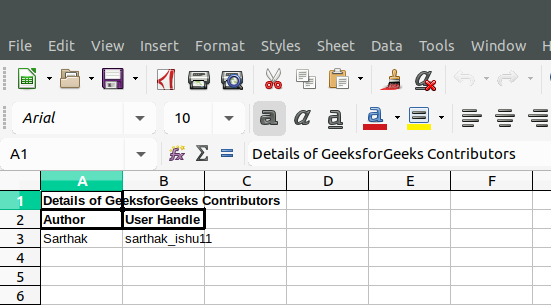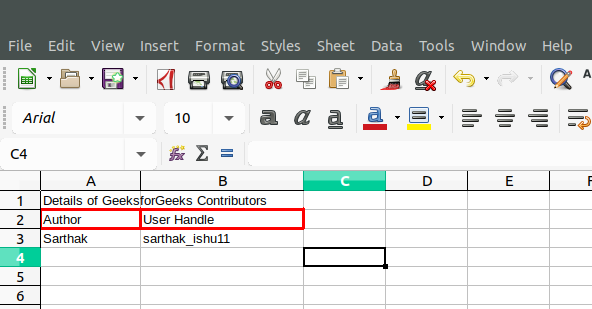Open in App
Not now

• Difficulty Level : Hard
• Last Updated : 04 Oct, 2021

The addWorksheet() function is an inbuilt function in PHP | Spreadsheet_Excel_Writer which is used to add a new worksheet to the Excel workbook.

Syntax:

`Workbook::&addWorksheet( \$name='' )`

Parameters: This function accepts single parameter \$string as worksheet name which can’t be greater than 31 characters.

Return Value: This function returns reference to a worksheet object on success and PEAR_ERROR on failure.

Example 1:

## PHP

 `addFormat();`   `// Set Bold Format ` `\$format_bold``->setBold();`   `// Add Worksheet to Spreadsheet` `\$worksheet` `=& ``\$workbook``->addWorksheet();`   `\$format_bold``->setBorder(2);`   `// Write to Worksheet` `\$worksheet``->write(0, 0, "Details of GeeksforGeeks ` `                      ``Contributors", ``\$format_bold``);` `\$worksheet``->write(1, 0, ``"Author"``, ``\$format_bold``);` `\$worksheet``->write(1, 1, ``"User Handle"``, ``\$format_bold``);` `\$worksheet``->write(2, 0, ``"Sarthak"``);` `\$worksheet``->write(2, 1, ``"sarthak_ishu11"``);`   `// Send .xlsx file to header` `\$workbook``->send(``'test.xls'``);`   `// Close Workbook Object` `\$workbook``->close();` `?>`

Output:Example 2:

## PHP

 `addFormat();`   `// Add Worksheet to Spreadsheet` `\$worksheet` `=& ``\$workbook``->addWorksheet();`   `// Set and Add Border Width` `\$format_border``->setBorder(2);`   `// Set and Add Color to Border` `\$format_border``->setBorderColor(``'red'``);`   `// Write to Worksheet` `\$worksheet``->write(0, 0, "Details of GeeksforGeeks ` `                     ``Contributors", ``\$format_bold``);` `\$worksheet``->write(1, 0, ``"Author"``, ``\$format_border``);` `\$worksheet``->write(1, 1, ``"User Handle"``, ``\$format_border``);` `\$worksheet``->write(2, 0, ``"Sarthak"``);` `\$worksheet``->write(2, 1, ``"sarthak_ishu11"``);`   `// Send .xlsx file to header` `\$workbook``->send(``'test.xls'``);`   `// Close Workbook Object` `\$workbook``->close();` `?>`

Output:My Personal Notes arrow_drop_up
Related Articles